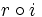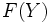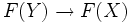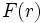# Retraction

## Definition

### Symbol-free definition

A retraction of a topological space is an idempotent continuous map from the topological space to itself. In other words, it is a continuous map from the topological space to a subspace, such that the restriction to that subspace is the identity map.

The image of a retraction is termed a retract (this is a subspace property).

### Definition with symbols

Fill this in later

## Facts

Given any functor from the category of topological spaces to the category of groups, or the category of modules over a ring, the topological space notion of retract gets mapped to the notion of retract in the relevant category.

Let$F$ be a functor from the category of topological spaces to the category of groups.

Suppose$X$ is a topological space,$r$ is a retraction and$Y$ is the image of the retraction. Suppose$i$ is the inclusion of$Y$ in$X$. Since$r \circ i$ is the identity map on$Y$, we get by functorality that$F(r) \circ F(i)$ is the identity on$F(Y)$. This forces that$F(Y) \to F(X)$ is injective, and$F(X) \to F(Y)$ is surjective. Identifying$F(Y)$ with its image subgroup$F(X)$, we can view$F(r)$ as a retraction of groups.

The above can be applied to the fundamental group, any of the higher homotopy groups, or any of the homology groups.